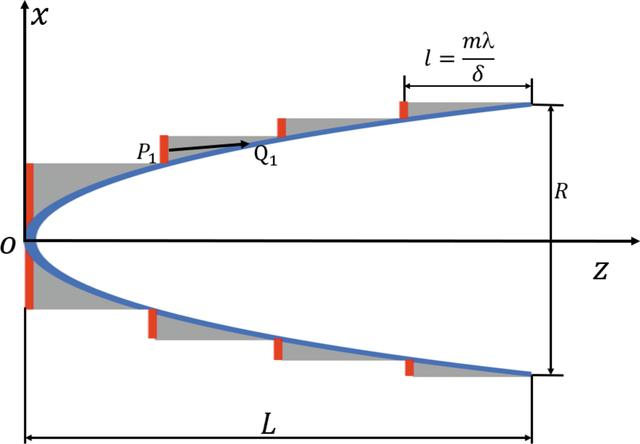disable zoom     view article Figure 1 Schematic diagram of a long kinoform lens. The x and z axes denote the transversal and longitudinal directions, L denotes the total length of the lens, R denotes the aperture of the lens, l denotes the length of each step, and P1 and Q1 denote the points at the incident and exit surfaces, respectively.JOURNAL OFSYNCHROTRONRADIATION
ISSN: 1600-5775
Volume 29| Part 6| November 2022| Pages 1338-1343